# Getting Started with dssd

Distance sampling survey design.

Laura Marshall http://distancesampling.org (CREEM, Univ of St Andrews)https://creem.st-andrews.ac.uk
2020-02-02

## Survey Design for Distance Sampling

Distance Sampling techniques provide design based estimates of density and abundance for populations, the accuracy of these estimates therefore relies on valid survey design. The process of designing a survey involves deciding on the required survey effort to achieve a desired precision followed by deciding where exactly the effort (the lines or points) will be located within the survey region.

Before proceeding to the design stage there are a number of considerations which first need to be addressed. The first steps in conducting a survey are to clearly define the study population and the objectives of the study. In addition, the use of spatial stratification to improve precision should also be considered, the Multiple Strata in dssd vignette details how to work with multi-strata study regions. We recommend consulting (Buckland, Anderson, Burnham, Borchers, & Thomas, 2001) and (Strindberg, Buckland, & Thomas, 2004) before using dssd to design your distance sampling survey.

Once you have defined your population and study objectives, and estimated the required effort, dssd (Marshall, 2019a) can help ensure you meet the survey design assumptions associated with distance sampling. Distance sampling assumes that the survey transects are a representative sample of the study region and are laid out at random with respect to the population. dssd achieves both these objectives by randomising the locations of the transects. Designs may be fully random with each transect being randomly located independently of other transects or a systematic design with a random start point should also meet this assumption.

The next assumption is that each point within the study region is equally likely to be sampled as any other, we call this uniform coverage. We can assess the coverage across the study region using a grid of points called a coverage grid. Some designs have more uniform coverage than others and dssd can help assess how uniform the coverage is via simulation. If non-uniform coverage is of concern dssd can be used in conjunction with DSsim (Marshall, 2019b) the distance sampling simulations package to better assess the effects of non-uniform coverage under normal and worst case scenarios to allow a more informed choice of design.

## Setting up the Region

The first step in creating a survey design is to set up your study region. The easiest way to do this is using a shapefile which has already been created to define your study region. The dssd package contains the example shapefile used in this vignette and can be accessed using the code below. Here we use this shapefile to define the study region and then plot it.


library(dssd)
shapefile.name <- system.file("extdata", "TrackExample.shp", package = "dssd")
region <- make.region(region.name = "study area",
shape = shapefile.name)
plot(region)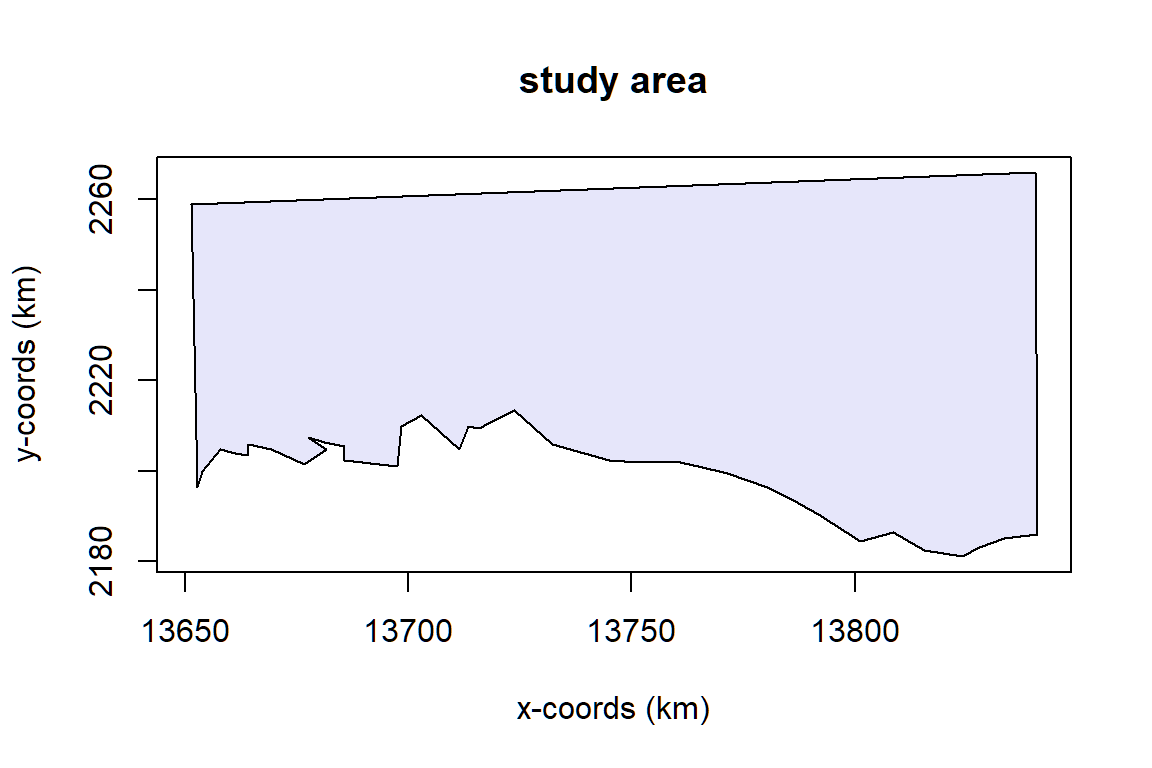Figure 1: The study region.

## Creating a Coverage Grid

Ideally, a coverage grid is created separately before creating the design. The same coverage grid can then be used for multiple designs. If a coverage grid is not passed to the design then this will be detected when the coverage simulation is run and a grid with 1000 points will automatically be generated.


cover <- make.coverage(region,
n.grid.points = 1000)

## Defining the Design

dssd provides a number of point and line transect designs. The design refers to a description of how the transect locations are selected and does not specify the locations of a single set of transects. We will later refer to a single set of transects generated from the design as a survey. A survey can then be thought if as a single random realisation of a design.

Here we will demonstrate a systematic parallel line design. We will define the desired effort as 1300 km. Note that the line length units will always be the same as the region coordinate units. Usually edge effects for line transect surveys are minimal so we will choose to carry out minus sampling, when only transects within the survey region are sampled. The design angle for parallel line designs is 0 for vertical lines and then moves round in a clockwise direction. Here I have left the design angle as 0 as this maximises the number of transects, the study region is larger in the x-dimension than the y-dimension. I have set the truncation distance to be 2 km, this is the maximum distance at which it is possible to make detections and is used to define the covered area. The covered area refers to the area of the study region which is sampled during the survey, this is a set of rectangles around line transects or a set of circles around point transects which are then clipped to the strata boundaries. If these rectangles or circles overlap, then the areas of overlap are counted twice. This means that it is possible, with a sufficiently large truncation distance, to sample more than 100% of the survey region.


design <- make.design(region = region,
design = "systematic",
line.length = 1300,
edge.protocol = "minus",
design.angle = 0,
truncation = 2,
coverage.grid = cover)

## Generating a Survey

Now we have defined the design we should check that it generated transects as we would expect by generating a survey. You can then view statistics about this survey and plot the transects.


transects <- generate.transects(design)
transects

Strata study area:
___________________
Design:  systematically spaced parallel transects
Spacing:  9.258935
Line length: 1346.139
Trackline length: 1536.667
Cyclic trackline length: 1722.431
Number of samplers:  21
Design angle:  0
Edge protocol:  minus
Covered area:  5282.704
Strata coverage: 43.89%
Strata area:  12036.62

Study Area Totals:
_________________
Line length: 1346.139
Trackline length: 1536.667
Cyclic trackline length: 1722.431
Number of samplers:  21
Covered area:  5282.704
Average coverage: 43.89%

plot(region, transects)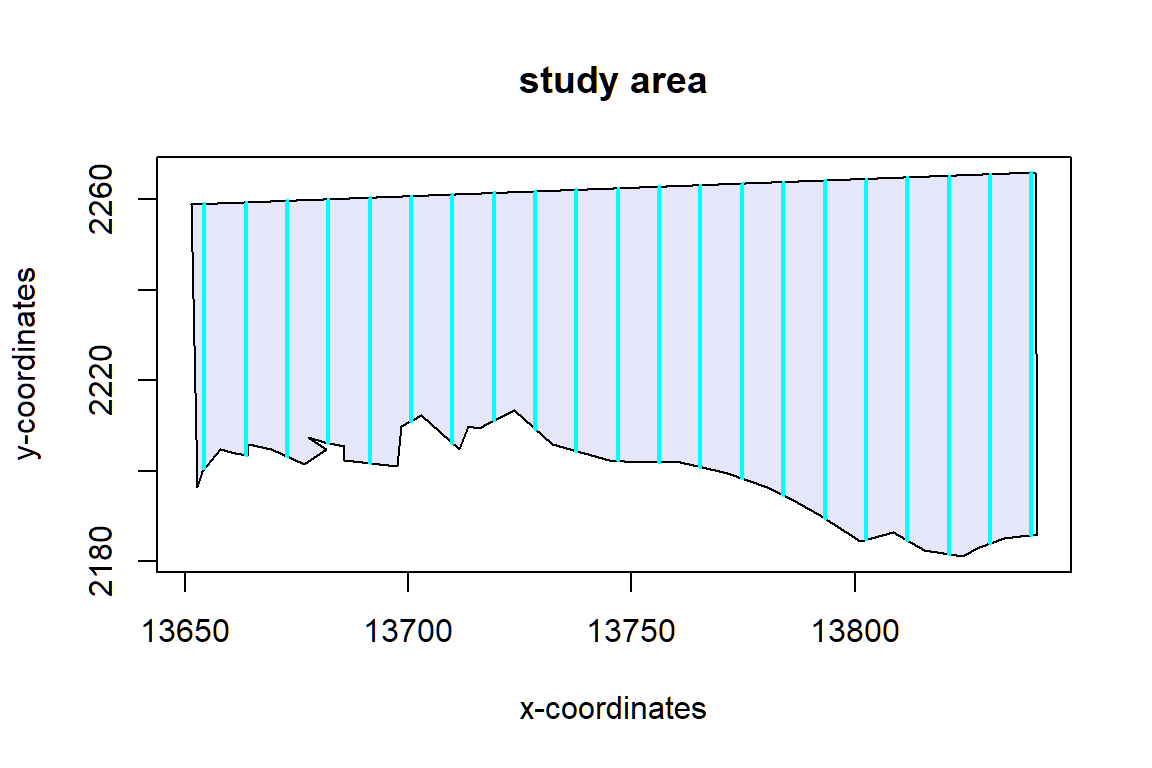Figure 2: An example set of transects generated from the design plotted within the study region.

We can see that this survey has an on effort line length of 1346 km, close to the 1300 km we asked for. It was generated based on a spacing of 9.26 km, a value calculated based on the requested line length, and resulted in 21 transects. We are also given the trackline length and cyclic trackline lengths. The trackline length is the sum of the lengths of the transects plus the off-effort transit time required to complete the survey from the beginning of the first transect to the end of the last transect. The off-effort transit distance is calculated as the crow flies and may be longer in reality if transit is required around lakes, islands or coastlines etc. The cyclic trackline length is the trackline length plus the off-effort transit distance required to return from the end of the last transect to the beginning of the first transect. Please see the appendix for more details and figures.

We are also told that this survey covers 43.9% of the study area. If we wish to view the covered area for this particular set of transects we can plot it using the code below. The covered area is the rectangles delineated by the black lines.


plot(region, transects, covered.area = TRUE)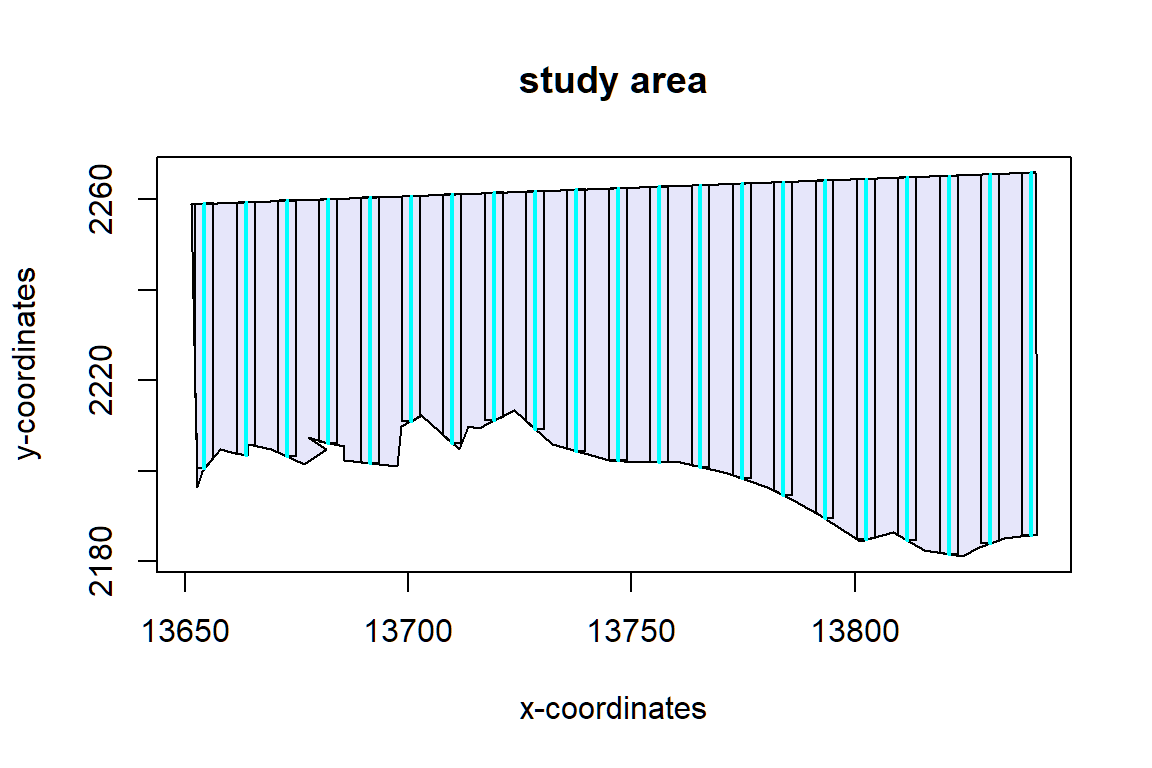Figure 3: An example set of transects generated from the design along with the covered area shown by the black rectangles around the transects.

## Assessing Coverage Uniformity and Design Statistics

Once we have checked that our design appears to be correctly specified we can assess how uniform the coverage is and also how values such as total line length, trackline length and cyclic trackline length vary across many surveys generated from our design. While we were given single values for these statistics from our one survey above it is important to check that all potential surveys from our design can be completed in the effort available, i.e. the maximum trackline/cyclic trackline length from the simulation should be achievable within the limits of the study. It is not permissible to generate more than one set of transects for the final survey and select the set with shorter/longer trackline length as then the selection of transect locations will no longer be purely random.

Parallel line designs should give uniform coverage across the majority of the survey region. The only exception to this is around the edge of the study region when we are using minus sampling, as we are using here. We will assess the effects of this now via a simulation which will generate 999 sets of transects from our design.


# This code will run the coverage simulations - it will take some time to complete
design <- run.coverage(design, reps = 999)

We can now view the coverage scores by plotting our design object.


plot(design, subtitle = "Systematic Parallel, line.length = 1300km")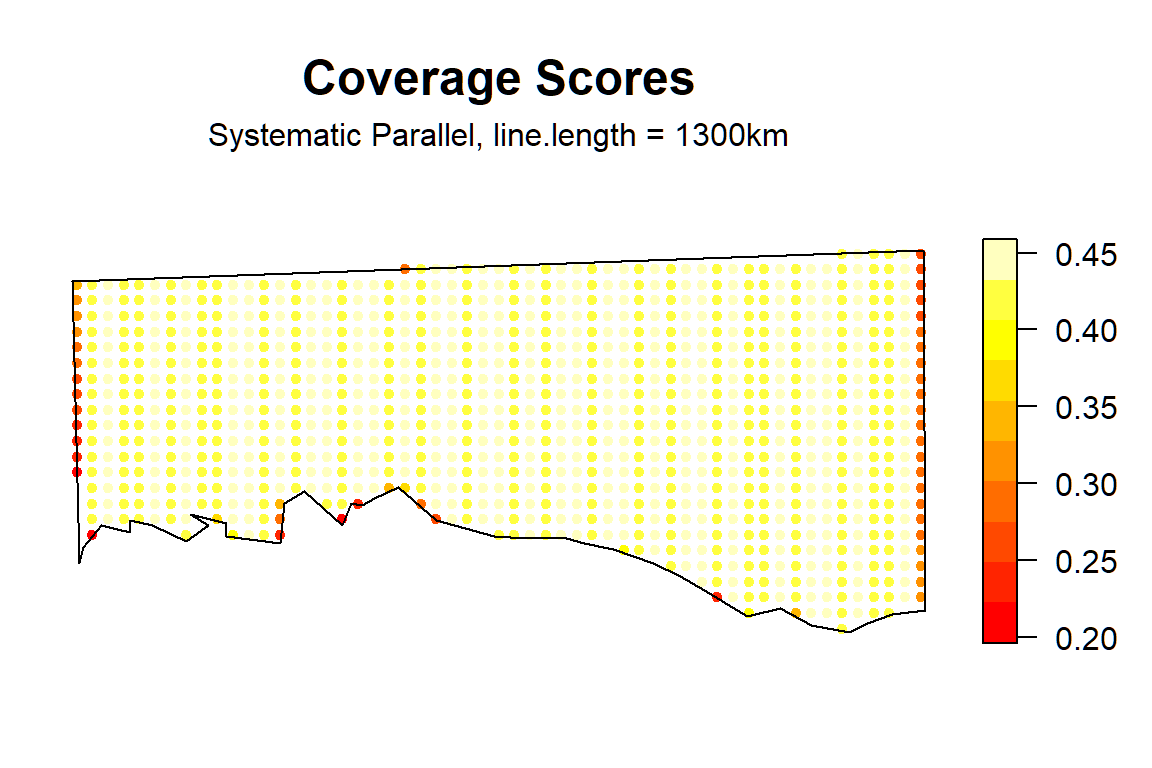Figure 4: The coverage grid

The plot above indicates that coverage is fairly uniform over the majority of the study region. There are a few points around the edge of the survey region which have lower coverage than the others due to the minus sampling protocol, however for this example we would expect the effects of non-uniform coverage on the study estimates to be very small. The coverage grid points with lower coverage are very few in number and represent a very small proportion of the study region area.

We can also look at the design statistics, these are shown below.


design

Strata study area:
___________________
Design:  systematically spaced transects
Spacing:  NA
Number of samplers:  NA
Line length:  1300  (shared across strata)
Design angle:  0
Edge protocol:  minus

Strata areas:  12037
Region and effort units:  km
Coverage Simulation repetitions:  999

Number of samplers:

study area Total
Minimum       20.0  20.0
Mean          20.5  20.5
Median        20.0  20.0
Maximum       21.0  21.0
sd             0.5   0.5

Covered area:

study area   Total
Minimum    5050.77 5050.77
Mean       5147.14 5147.14
Median     5101.63 5101.63
Maximum    5310.94 5310.94
sd           90.43   90.43

% of region covered:

study area Total
Minimum      41.96 41.96
Mean         42.76 42.76
Median       42.38 42.38
Maximum      44.12 44.12
sd            0.75  0.75

Line length:

study area   Total
Minimum    1268.02 1268.02
Mean       1299.52 1299.52
Median     1282.34 1282.34
Maximum    1348.13 1348.13
sd           31.17   31.17

Trackline length:

study area   Total
Minimum    1450.50 1450.50
Mean       1485.09 1485.09
Median     1463.06 1463.06
Maximum    1538.98 1538.98
sd           35.32   35.32

Cyclic trackline length:

study area   Total
Minimum    1637.21 1637.21
Mean       1672.06 1672.06
Median     1649.37 1649.37
Maximum    1724.80 1724.80
sd           35.20   35.20

Coverage Score Summary:

study area      Total
Minimum 0.19619620 0.19619620
Mean    0.42611584 0.42611584
Median  0.43243243 0.43243243
Maximum 0.45845846 0.45845846
sd      0.03585962 0.03585962

First you are given a summary of the design, systematically spaced transects where the spacing was to be selected to achieve around 1300 km of effort. The lines were to be placed on a design angle of 0 and a minus sampling protocol was to be used.

We are then told the area of the study region, the units of the region coordinates and how many times the coverage simulation was repeated.

We now move on to the summary statistics from the 999 sets of transects generated during the simulation. Note if you re-ran the simulation then your results may differ slightly as they will be based on a different 999 sets of transects.

We are told that each survey has between 20 and 21 samplers and that the minimum and maximum covered areas are 5051 and 5311 km2 which equate to 42.0% and 44.1% of the study area, respectively. The minimum on-effort line length is 1268 km and the maximum on-effort line length is 1348 km, with a mean value of very close to the 1300km requested. We are then given the minimum, mean, median, maximum and standard deviation values for the trackline and cyclic trackline lengths. The maximum values for these statistics should be used to ensure that any set of transects randomly generated from the design is achievable in the total effort available given any time and financial constraints of the survey.

Finally, we are given a summary of the coverage score values. If we have even coverage then we should see little variation in the coverage scores. The minimum coverage score is 0.20 and the maximum is 0.46 indicating that some small areas around the edge of the study region (as shown in Figure 4) are half as likely to be sampled as those within the main part of the study region. We are given an indication of the variability of the coverage scores in the standard deviation provided but it may also be useful to plot a histogram of the coverage scores, Figure 5. Again this looks fairly reassuring that the parts of the study with lower coverage do not represent a significant proportion of the study area. However, if we wanted to be really thorough we could run a simulation study using DSsim and test a worse case scenario, where density in these areas of lower coverage varied from the rest of the study region. If we were worried that this non-uniform coverage could cause significant bias we could switch to a plus sampling strategy.


hist(get.coverage(design), xlab = "Coverage Scores", main = "Histogram of Coverage Scores")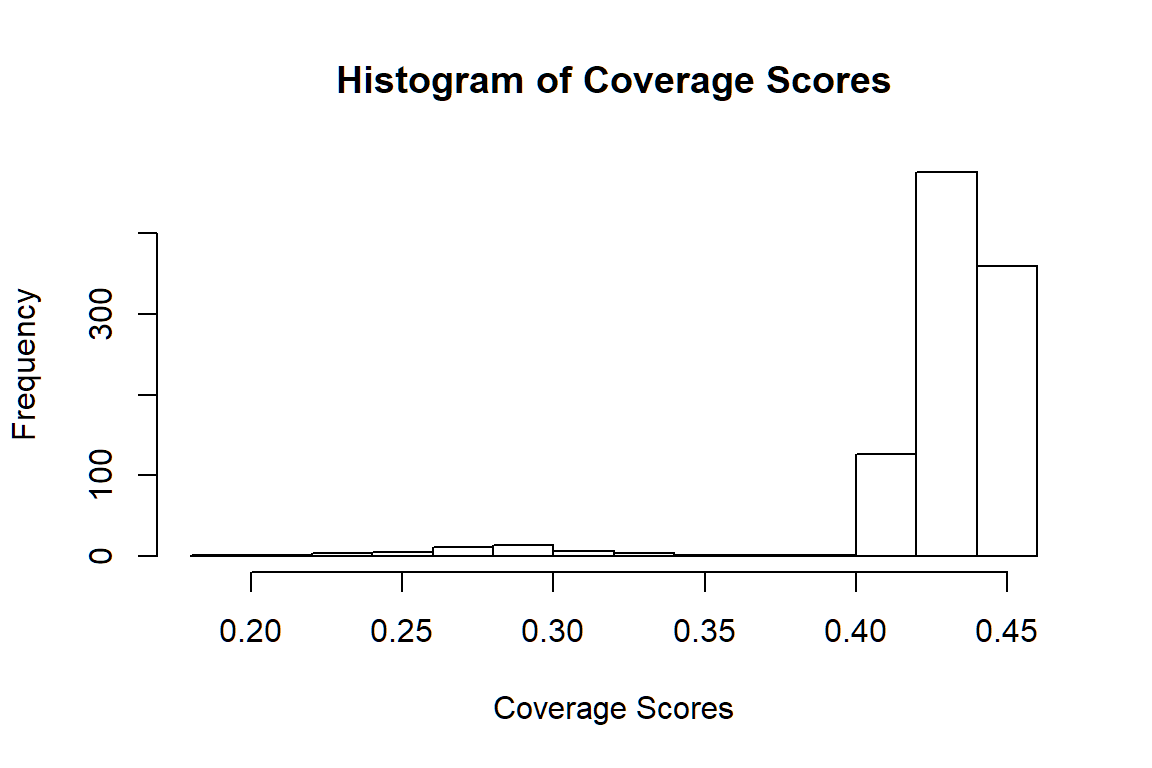Figure 5: Histogram of coverage scores.

## Appendix: Trackline and Cyclic Trackline Lengths

The following figures demonstrate how the trackline lengths and cyclic trackline lengths are calculated. The red arrows indicate the trackline path, moving from the start of the first transect along its length then across off effort to the next transect and so on until the end of the last transect is reached. The cyclic trackline length is then the trackline length represented by the red arrows plus the off effort transit time required to travel from the end of the last transect back to the beginning of the first as indicated by the light blue arrow. These values are provided to help you assess the efficiency of the design by comparing on-effort line length to total trackline length and also to help you ensure that the entire survey can be completed within time and budget constraints. Note that the trackline and cyclic trackline lengths for segmented designs are calculated in a very similar way to parallel line transect designs. The observer will start at the beginning of one segment and move up the line of segments before crossing to the next line of segments and so on.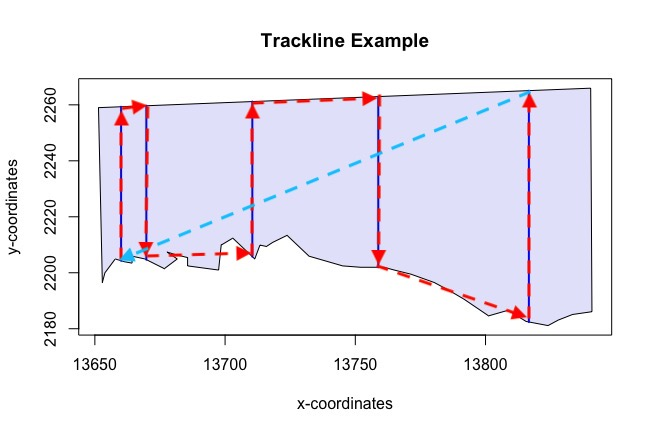Figure 6: Illustrates the trackline and cyclic trackline lengths for random parallel line designs. The sum of the lengths of the red arrows represent the trackline length. The sum of the lengths of the red arrows and the light blue arrow represent the cyclic trackline length.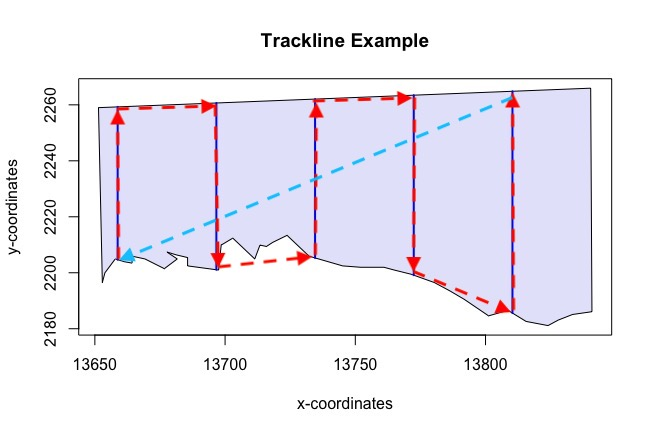Figure 7: Illustrates the trackline and cyclic trackline lengths for systematic parallel line designs. The sum of the lengths of the red arrows represent the trackline length. The sum of the lengths of the red arrows and the light blue arrow represent the cyclic trackline length.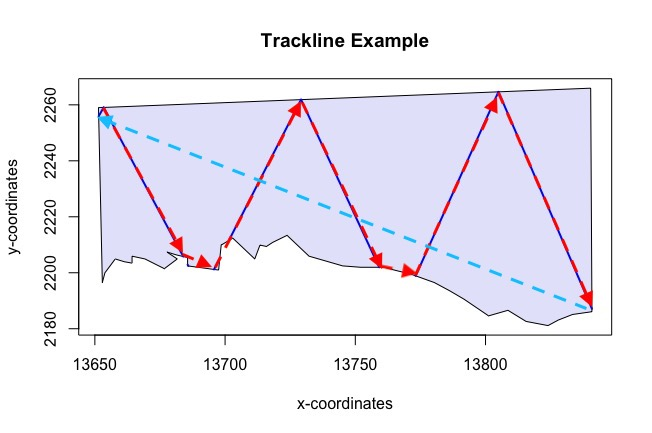Figure 8: Illustrates the trackline and cyclic trackline lengths for zigzag designs. The sum of the lengths of the red arrows represent the trackline length. The sum of the lengths of the red arrows and the light blue arrow represent the cyclic trackline length.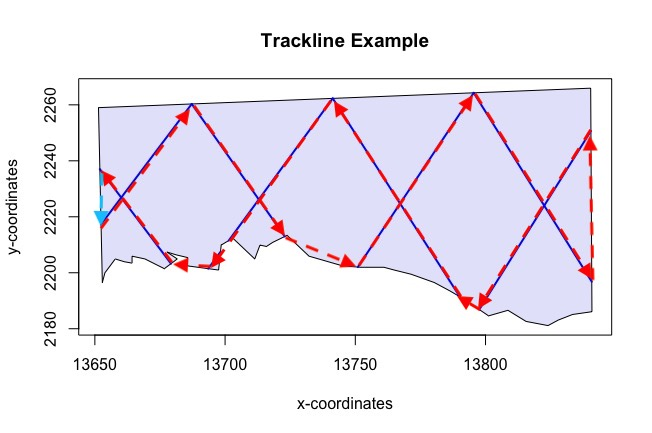Figure 9: Illustrates the trackline and cyclic trackline lengths for complementary zigzag designs. The sum of the lengths of the red arrows represent the trackline length. The sum of the lengths of the red arrows and the light blue arrow represent the cyclic trackline length.

Buckland, S. T., Anderson, D. R., Burnham, K. P., Borchers, D. L., & Thomas, L. (2001). Introduction to distance sampling. Oxford University Press, Oxford, UK.